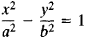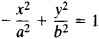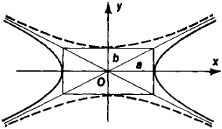# Conjugate Hyperbolas

## conjugate hyperbolas

[′kän·jə·gət hī′pər·bə·ləz]
(mathematics)
Two hyperbolas having the same asymptotes with semiaxes interchanged.
McGraw-Hill Dictionary of Scientific & Technical Terms, 6E, Copyright © 2003 by The McGraw-Hill Companies, Inc.
The following article is from The Great Soviet Encyclopedia (1979). It might be outdated or ideologically biased.

## Conjugate Hyperbolas

two hyperbolas defined in the same rectangular coordinate system by the equationsandwhere a and b have the same values in both equations. Conjugate hyperbolas have the same asymptotes. The transverse and conju-Figure 1

gate axes of one conjugate hyperbola are, respectively, the conjugate and transverse axes of the other (see Figure I).

The Great Soviet Encyclopedia, 3rd Edition (1970-1979). © 2010 The Gale Group, Inc. All rights reserved.
Site: Follow: Share:
Open / Close